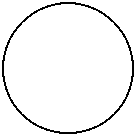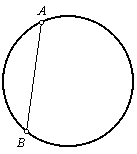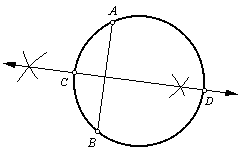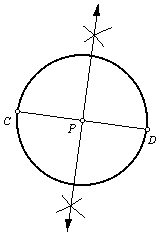Construct the center point of a given circle.

 1. Begin with a circle, but no center point.2. Draw chord AB.3. Construct the perpendicular bisector of chord AB. Let C and D be the points where it intersects the circle. (Refer to the construction of a perpendicular bisector.)4. Chord CD is a diameter of the circle. Construct point P, the midpoint of diameter CD. Point P is the center point of the circle. (Refer to the construction of a the midpoint of a line segment.)